summary of site-wide JavaScript functionalityUnited States-English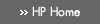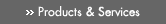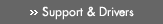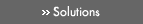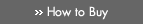» Contact HPSearch:More optionsManualTechnical documentation - EnglishAll of HP USHP-UX Reference > R# rsqrt(3M)

HP Integrity Server Only
HP-UX 11i Version 3: February 2007»

## Technical documentation» Feedback## » Index## NAME

rsqrt(), rsqrtf(), rsqrtl(), rsqrtw(), rsqrtq() — reciprocal square root functions

## SYNOPSIS

#include <math.h>

double rsqrt(double x);

float rsqrtf(float x);

long double rsqrtl(long double x);

extended rsqrtw(extended x);

## DESCRIPTION

These functions are available only for Integrity servers.

rsqrt() returns the reciprocal of the non-negative square root of x.

The expression a*rsqrt(b) is intended as a higher performance alternative to a/sqrt(b). The two expressions are comparably accurate, but do not compute exactly the same value in every case. For example, a*rsqrt(a*a + b*b) can be just slightly greater than 1, in rare cases.

rsqrtf() is a float version of rsqrt(); it takes a float argument and returns a float result.

### Integrity Server Only

rsqrtl() is a long double version of rsqrt(); it takes a long double argument and returns a long double result.

rsqrtw() is an extended version of rsqrt(); it takes an extended argument and returns an extended result.

rsqrtq() is equivalent to rsqrtl() on HP-UX systems.

## USAGE

To use these functions compile either with the default -Ae option or with the -Aa and the -D_HPUX_SOURCE options.

To use (for Integrity servers) rsqrtw() or rsqrtq(), compile also with the -fpwidetypes option.

To use any of these functions, make sure your program includes <math.h>, and link in the math library by specifying -lm on the compiler or linker command line.

## RETURN VALUE

If x is ±0, rsqrt() returns ±INFINITY and raises the divide-by-zero exception.

If x is +INFINITY, rsqrt() returns +0.

If x is NaN, rsqrt() returns NaN.

If x is less than zero, rsqrt() returns NaN and raises the invalid floating-point exception.

rsqrt() raises the inexact exception whenever a rounded result does not equal the mathematical result.

## ERRORS

No errors are defined.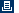Printable versionPrivacy statement Using this site means you accept its terms Feedback to webmaster© 1983-2007 Hewlett-Packard Development Company, L.P.